Examples¶

Simple phase¶

This example illustrates the simple usage of the qpimage.QPImage class for reading and managing quantitative phase data. The attribute QPImage.pha yields the background-corrected phase data and the attribute QPImage.bg_pha yields the background phase image.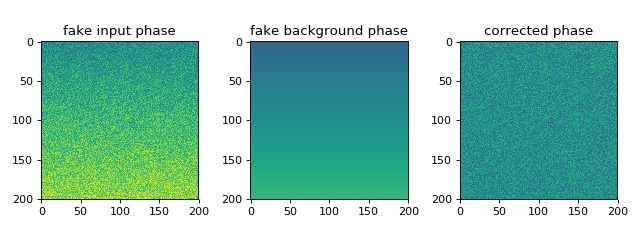simple_phase.py

 1 2 3 4 5 6 7 8 9 10 11 12 13 14 15 16 17 18 19 20 21 22 23 24 25 26 27 28 29 import matplotlib.pylab as plt import numpy as np import qpimage size = 200 # background phase image with a tilt bg = np.repeat(np.linspace(0, 1, size), size).reshape(size, size) # phase image with random noise phase = np.random.rand(size, size) + bg # create QPImage instance qpi = qpimage.QPImage(data=phase, bg_data=bg, which_data="phase") # plot the properties of `qpi` plt.figure(figsize=(8, 3)) plot_kw = {"vmin": -1, "vmax": 2} plt.subplot(131, title="fake input phase") plt.imshow(phase, **plot_kw) plt.subplot(132, title="fake background phase") plt.imshow(qpi.bg_pha, **plot_kw) plt.subplot(133, title="corrected phase") plt.imshow(qpi.pha, **plot_kw) plt.tight_layout() plt.show()

Background image tilt correction¶

This example illustrates background tilt correction with qpimage. In contrast to the ‘simple_phase.py’ example, the known background data is not given to the qpimage.QPImage class. In this particular example, the background tilt correction achieves an error of about 1% which is sufficient in most quantitative phase imaging applications.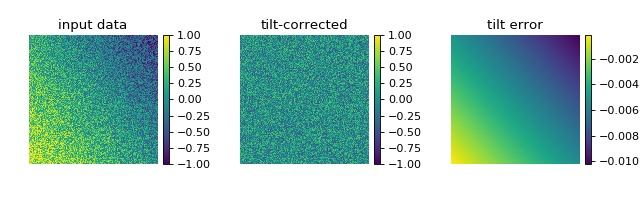background_tilt.py

 1 2 3 4 5 6 7 8 9 10 11 12 13 14 15 16 17 18 19 20 21 22 23 24 25 26 27 28 29 30 31 32 33 34 35 36 37 38 39 40 41 42 43 import matplotlib.pylab as plt import numpy as np import qpimage size = 200 # background phase image with a tilt bg = np.repeat(np.linspace(0, 1, size), size).reshape(size, size) bg = .6 * bg - .8 * bg.transpose() + .2 # phase image with random noise rsobj = np.random.RandomState(47) phase = rsobj.rand(size, size) - .5 + bg # create QPImage instance qpi = qpimage.QPImage(data=phase, which_data="phase") # compute background with 2d tilt approach qpi.compute_bg(which_data="phase", # correct phase image fit_offset="fit", # use bg offset from tilt fit fit_profile="tilt", # perform 2D tilt fit border_px=5, # use 5 px border around image ) # plot the properties of `qpi` fig = plt.figure(figsize=(8, 2.5)) plot_kw = {"vmin": -1, "vmax": 1} ax1 = plt.subplot(131, title="input data") map1 = ax1.imshow(phase, **plot_kw) plt.colorbar(map1, ax=ax1, fraction=.046, pad=0.04) ax2 = plt.subplot(132, title="tilt-corrected") map2 = ax2.imshow(qpi.pha, **plot_kw) plt.colorbar(map2, ax=ax2, fraction=.046, pad=0.04) ax3 = plt.subplot(133, title="tilt error") map3 = ax3.imshow(bg - qpi.bg_pha) plt.colorbar(map3, ax=ax3, fraction=.046, pad=0.04) # disable axes [ax.axis("off") for ax in [ax1, ax2, ax3]] plt.tight_layout(pad=0, h_pad=0, w_pad=0) plt.show()

Background image offset correction¶

This example illustrates the different background offset correction methods implemented in qpimage. The phase image data contains two gaussian noise distributions for which these methods yield different background phase offsets.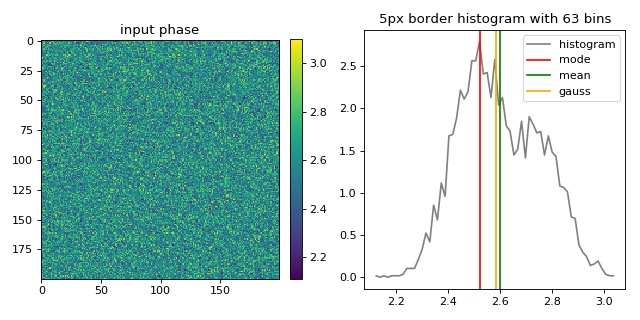background_offset.py

 1 2 3 4 5 6 7 8 9 10 11 12 13 14 15 16 17 18 19 20 21 22 23 24 25 26 27 28 29 30 31 32 33 34 35 36 37 38 39 40 41 42 43 44 45 46 47 48 49 50 51 52 53 54 55 56 57 58 59 60 61 62 63 64 import matplotlib.pylab as plt import numpy as np import qpimage size = 200 # the size of the image bg = 2.5 # the center of the background phase distribution scale = .1 # the spread of the background phase distribution # compute random phase data rsobj = np.random.RandomState(42) data = rsobj.normal(loc=bg, scale=scale, size=size**2) # Add a second distribution `data2` at random positions `idx`, # such that there is no pure gaussian distribution. # (otherwise 'mean' and 'gaussian' cannot be distinguished) data2 = rsobj.normal(loc=bg*1.1, scale=scale, size=size**2//2) idx = rsobj.choice(data.size, data.size//2) data[idx] = data2 # reshape `data` to get a 2D array data = data.reshape(size, size) qpi = qpimage.QPImage(data=data, which_data="phase") cpkw = {"which_data": "phase", # correct the input phase data "fit_profile": "offset", # perform offset correction only "border_px": 5, # use a border of 5px of the input phase "ret_mask": True, # return the mask image for visualization } mask = qpi.compute_bg(fit_offset="mode", **cpkw) bg_mode = np.mean(qpi.bg_pha[mask]) qpi.compute_bg(fit_offset="mean", **cpkw) bg_mean = np.mean(qpi.bg_pha[mask]) qpi.compute_bg(fit_offset="gauss", **cpkw) bg_gauss = np.mean(qpi.bg_pha[mask]) bg_data = (qpi.pha + qpi.bg_pha)[mask] # compute histogram nbins = int(np.ceil(np.sqrt(bg_data.size))) mind, maxd = bg_data.min(), bg_data.max() histo = np.histogram(bg_data, nbins, density=True, range=(mind, maxd)) dx = abs(histo - histo) / 2 hx = histo[1:] - dx hy = histo # plot the properties of `qpi` plt.figure(figsize=(8, 4)) ax1 = plt.subplot(121, title="input phase") map1 = plt.imshow(data) plt.colorbar(map1, ax=ax1, fraction=.046, pad=0.04) t2 = "{}px border histogram with {} bins".format(cpkw["border_px"], nbins) plt.subplot(122, title=t2) plt.plot(hx, hy, label="histogram", color="gray") plt.axvline(bg_mode, 0, 1, label="mode", color="red") plt.axvline(bg_mean, 0, 1, label="mean", color="green") plt.axvline(bg_gauss, 0, 1, label="gauss", color="orange") plt.legend() plt.tight_layout() plt.show()

Masked background image correction¶

This example illustrates background correction with qpimage using a mask to exclude regions that do not contain background information.

The phase image of a microgel bead (top left) has two artifacts; there is a tilt-like phase profile added along the vertical axis and there is a second microgel bead in close proximity to the center bead. A regular phase tilt background correction using the image values around a frame of five pixels (see “background_tilt.py” example) does not yield a flat background, because the second bead is fitted into the background which leads to a horizontal background phase profile (top right). By defining a mask (bottom left image), the phase values of the second bead can be excluded from the background tilt fit and a flat background phase is achieved (bottom right).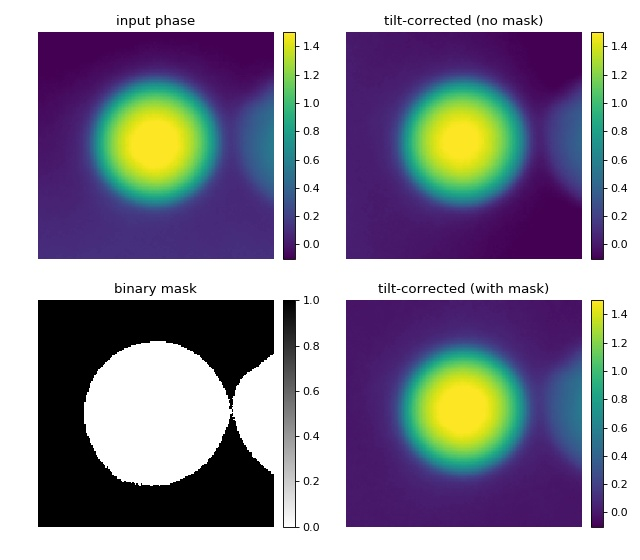Background image 2nd order polynomial correction¶

This example extends the tilt correction (‘background_tilt.py’) to a second order polynomial correction for samples that exhibit more sophisticated phase aberrations. The phase background correction is computed from a ten pixel wide frame around the image. The phase data shown are computed from a hologram of a single myeloid leukemia cell (HL60) recorded using digital holographic microscopy (DHM) (see [SSM+15]).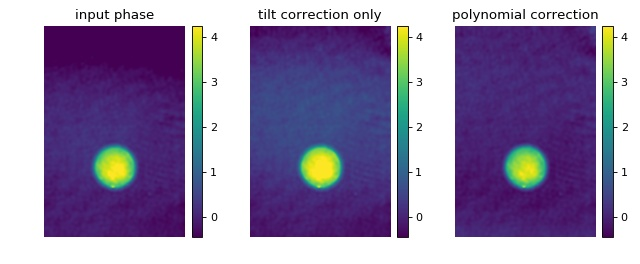background_poly2o.py

 1 2 3 4 5 6 7 8 9 10 11 12 13 14 15 16 17 18 19 20 21 22 23 24 25 26 27 28 29 30 31 32 33 34 35 36 37 38 39 40 41 42 43 44 45 46 47 48 49 50 51 52 53 54 import matplotlib.pylab as plt import numpy as np # The data are stored in a .jpg file (lossy compression). # If `PIL` is not found, try installing the `pillow` package. from PIL import Image import qpimage edata = np.array(Image.open("./data/hologram_cell_curved_bg.jpg")) # create QPImage instance qpi = qpimage.QPImage(data=edata, which_data="hologram") pha0 = qpi.pha # background correction using tilt only qpi.compute_bg(which_data=["phase"], fit_offset="fit", fit_profile="tilt", border_px=10, ) pha_tilt = qpi.pha # background correction using polynomial qpi.compute_bg(which_data=["phase"], fit_offset="fit", fit_profile="poly2o", border_px=10, ) pha_poly2o = qpi.pha # plot phase data fig = plt.figure(figsize=(8, 3.3)) phakw = {"cmap": "viridis", "interpolation": "bicubic", "vmin": pha_poly2o.min(), "vmax": pha_poly2o.max()} ax1 = plt.subplot(131, title="input phase") map1 = ax1.imshow(pha0, **phakw) plt.colorbar(map1, ax=ax1, fraction=.067, pad=0.04) ax2 = plt.subplot(132, title="tilt correction only") map2 = ax2.imshow(pha_tilt, **phakw) plt.colorbar(map2, ax=ax2, fraction=.067, pad=0.04) ax3 = plt.subplot(133, title="polynomial correction") map3 = ax3.imshow(pha_poly2o, **phakw) plt.colorbar(map3, ax=ax3, fraction=.067, pad=0.04) # disable axes [ax.axis("off") for ax in [ax1, ax2, ax3]] plt.tight_layout(w_pad=0) plt.show()

Object-mask background image correction¶

In some cases, using only the border of the phase image for background correction might not be enough. To increase the area of the background image, it is possible to mask only the cell area. The qpsphere package provides the convenience method qpsphere.cnvnc.bg_phase_mask_for_qpi() which computes the background phase mask based on the position and radius of an automatically detected spherical phase object. The sized of the mask can be tuned with the radial_clearance parameter.

Note that the various methods used in the examples for determining such a phase mask can be combined. Also note that before applying the method discussed here, an initial background correction might be necessary.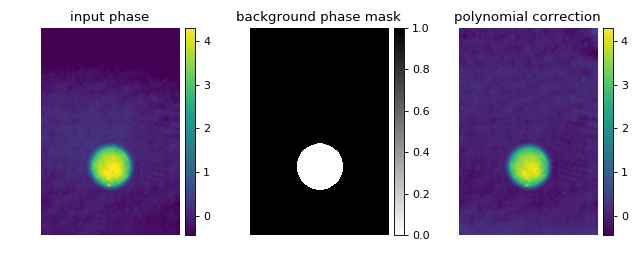Digital hologram of a single cell¶

This example illustrates how qpimage can be used to analyze digital holograms. The hologram of a single myeloid leukemia cell (HL60) shown was recorded using digital holographic microscopy (DHM). Because the phase-retrieval method used in DHM is based on the discrete Fourier transform, there always is a residual background phase tilt which must be removed for further image analysis. The setup used for recording these data is described in reference [SSM+15], which also contains a description of the hologram-to-phase conversion and phase background correction algorithms which qpimage is based on.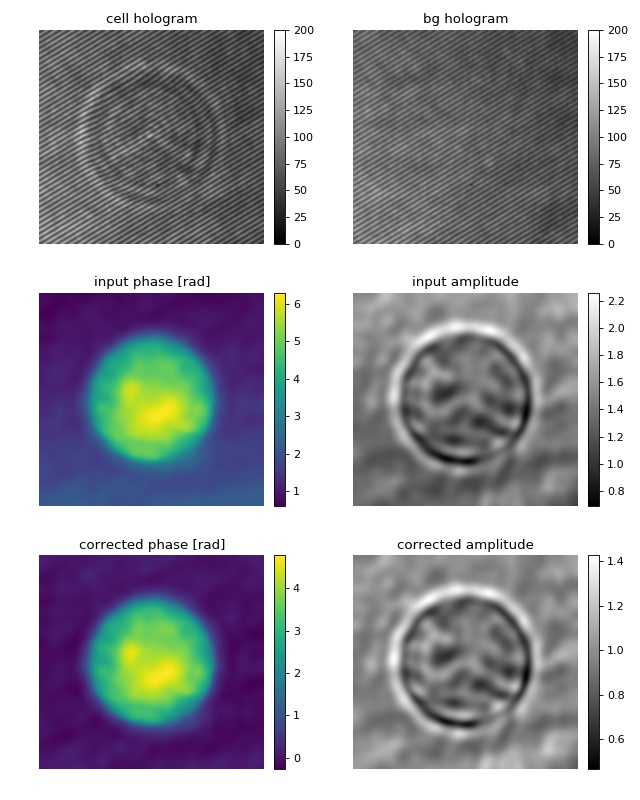hologram_cell.py

Hologram filter choice¶

There are several parameters that influence the quality of phase and amplitude data retrieved from holograms. This example demonstrates the advantages and disadvantages of a three hologram filters in qpimage. For more information, please have a look at qpimage.holo.get_field().

Several observations can be made:

• There appears to be a “bleed-through” of phase data into the amplitude data.
• A (sharp) disk filter introduces ringing artifacts in the amplitude and phase images.
• A smooth disk filter does not lead to such artifacts, but a dark halo is introduced around the coins in the amplitude image.
• The amplitude reconstruction with the gaussian filter does not exhibit the dark halo but, due to blurring, reveals less details.

To correctly interpret the data shown, please note that:

• This is a simulated hologram with no central band. For real data, the “filter_size” parameter also affects the reconstruction quality. Contributions from the central band can lead to strong artifacts. A balance between high resolution (large filter size) and small contributions from the central band (small filter size) usually has be found.
• It is not trivial to compare a gaussian filter with a disk filter in terms of filter size (sigma vs. radius). The gaussian filter takes into account larger frequencies and suppresses low frequencies. In qpimage, the actual gaussian filter size is chosen such that the resolution approximately matches that of the disk filter with a corresponding radius. In general however, the filter size parameter has to be examined when comparing the two.
• There is an inherent loss of information (resolution) in the holographic reconstruction process. The side band is isolated with a low-pass filter in Fourier space. The size and shape of this filter determine the resolution of the phase and amplitude images. As a result, the level of detail of all reconstructions shown is lower than that of the original images.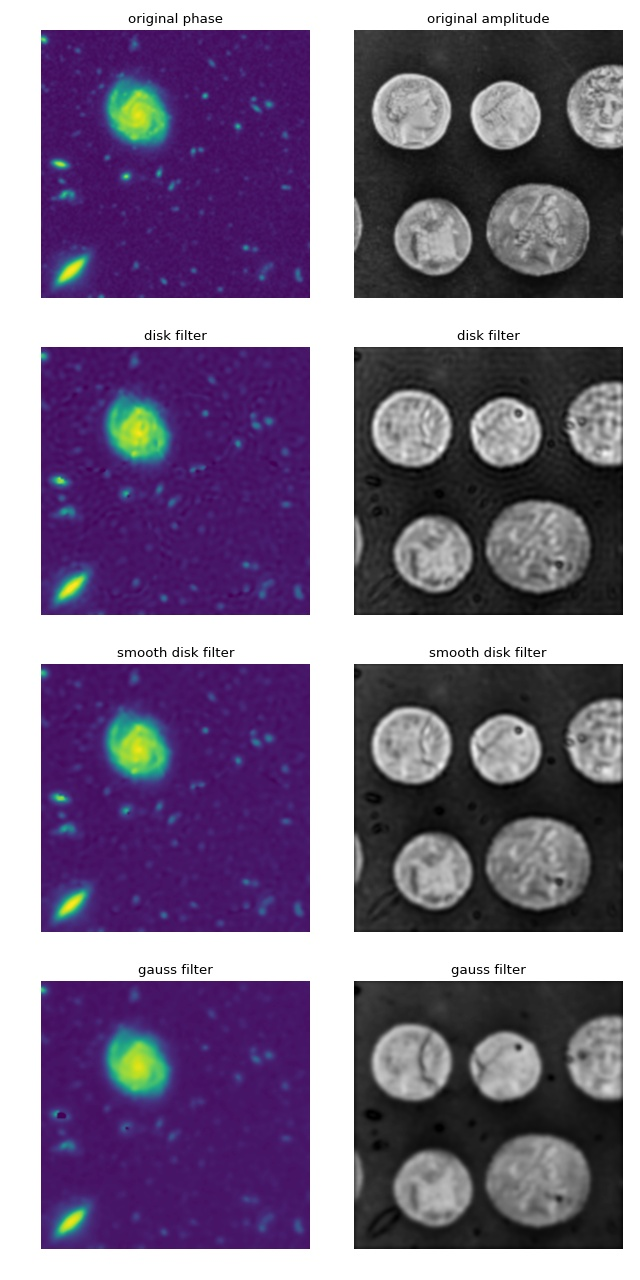hologram_filters.py

 1 2 3 4 5 6 7 8 9 10 11 12 13 14 15 16 17 18 19 20 21 22 23 24 25 26 27 28 29 30 31 32 33 34 35 36 37 38 39 40 41 42 43 44 45 46 47 48 49 50 51 52 53 54 55 56 57 58 59 60 61 62 63 import matplotlib.pylab as plt import numpy as np import qpimage from skimage import color, data # image of a galaxy recorded with the Hubble telescope img1 = color.rgb2grey(data.hubble_deep_field())[354:504, 70:220] # image of a coin img2 = color.rgb2grey(data.coins())[150:300, 70:220] pha = img1/img1.max() * 2 * np.pi amp = img2/img2.mean() # create a hologram x, y = np.mgrid[0:150, 0:150] hologram = 2 * amp * np.cos(-2 * (x + y) + pha) filters = ["disk", "smooth disk", "gauss"] qpis = [] for filter_name in filters: qpi = qpimage.QPImage(data=hologram, which_data="hologram", holo_kw={"filter_size": .5, "filter_name": filter_name}) qpis.append(qpi) fig = plt.figure(figsize=(8, 16)) phakw = {"interpolation": "bicubic", "cmap": "viridis", "vmin": pha.min(), "vmax": pha.max(), } ampkw = {"interpolation": "bicubic", "cmap": "gray", "vmin": amp.min(), "vmax": amp.max() } numrows = len(filters) + 1 plt.subplot(numrows, 2, 1, title="original phase") plt.imshow(pha, **phakw) ax2 = plt.subplot(numrows, 2, 2, title="original amplitude") plt.imshow(amp, **ampkw) for ii in range(len(filters)): # phase plt.subplot(numrows, 2, 2*ii+3, title=filters[ii]+" filter") plt.imshow(qpis[ii].pha, **phakw) # amplitude plt.subplot(numrows, 2, 2*ii+4, title=filters[ii]+" filter") plt.imshow(qpis[ii].amp, **ampkw) # disable axes for ax in fig.get_axes(): ax.axis("off") plt.tight_layout() plt.show()